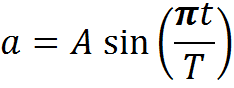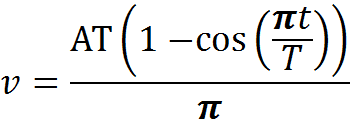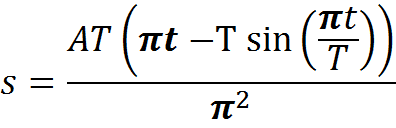# Movement with sine acceleration

• yaqwsx
In summary, The conversation is about finding the equation for velocity depending on track for a movement with sine acceleration. The equation for acceleration is provided and the speaker has integrated it to get an equation for velocity. However, they need to solve an equation in order to express t from the equation for track and use it in the equation for velocity. They are seeking help with finding a solution for this problem.

#### yaqwsx

Hello,
first of all, excuse my English. I have no experience witch describing mathematics problems in English.
I have a movement with sine acceleration. I need to get equation of velocity depending on track (I am not sure if that's the right expression in English - on the traveled distance).
Here's my equation for acceleration: a = A * sin(∏*t/T)where a is acceleration, A is the maximal acceleration, t is actual time and T is the whole time of accelerating. I want to get a velocity, so I integrated this expression and added parameter c to satisfy the condition: when t = 0, v = 0. I got this equation: v = (A*T/∏)*(1-cos(pi*t/T))If I wanted dependency veleocity on time, this would be enought, but I wanted depency velocity on track, so I have integrated... s = A*T*(∏*t - T*sin(∏*t/T))/∏^2I wanted to express t from this equation and put it into expression for velocity. For that I need to solve an equation in form a*x +sin(b*x) = c, which I can't solve. And as I have read it isn't something easy...
How can I get dependency of velocity on track for this movenent? I think there has to be a simple solution, but I don't see it. Can you help me, please?

can you just simply isolate AT from the equation for s and replace AT with it in the equation for v?

I think isolating AT won't help me - T is the whole time of accelerating - it's a constant. A is also a constnt. I need get rid of t - which is a variable and create dependency with s.